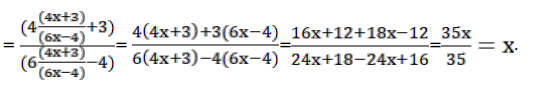# Solve thisQuestion:

If $f(x)=\frac{(4 x+3)}{(6 x-4)}, x \neq \frac{2}{3}$, show that (f o f) $(x)=x$ for all $x \neq \frac{2}{3}$

Hence, find $\mathrm{f}^{-1}$.

Solution:

To Show: that $f$ o $f(x)=x$

Finding (f o f) (x)